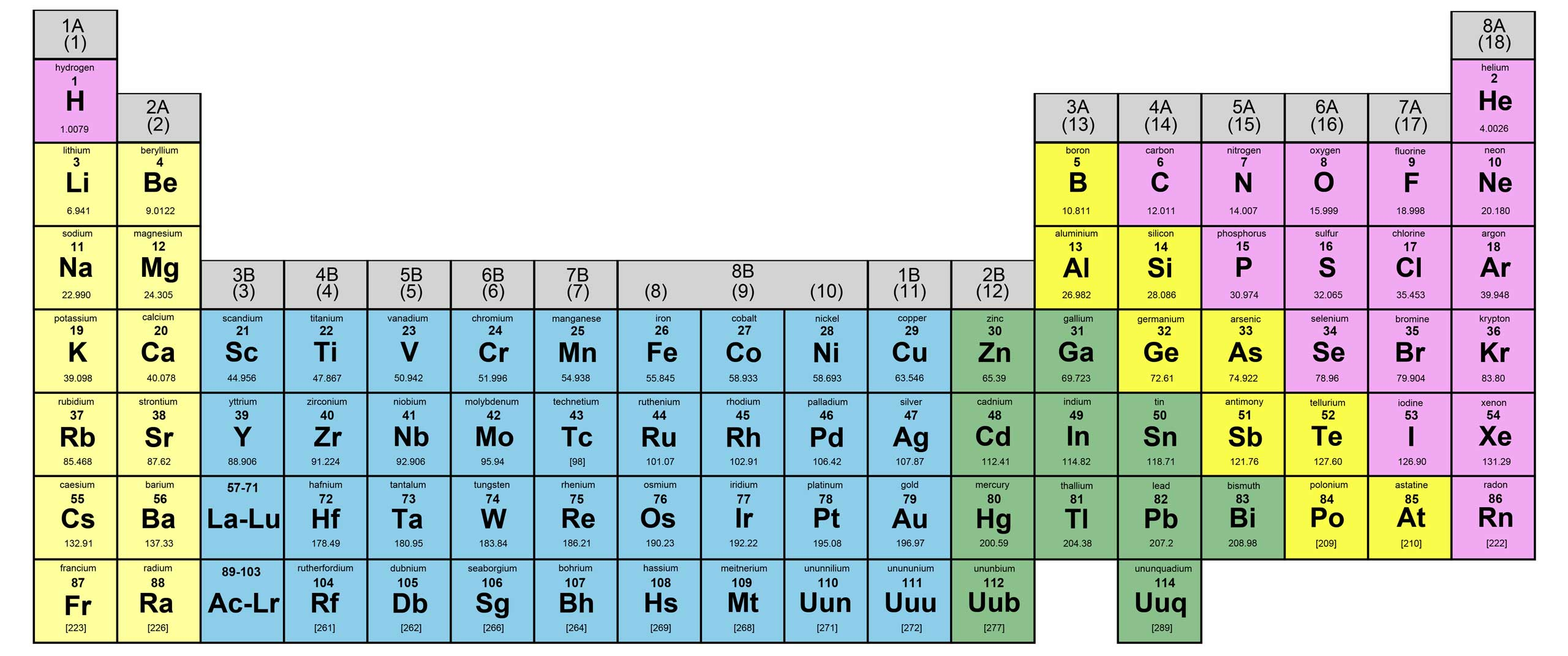Glossary:
 Qualitative Analysis The determination of what ions or elements are present in a material Quantization the concept that energy can occur only in discrete units called quanta Quantum A small, discrete unit of energy Quantum Numbers Numbers that govern the magnitude of quantized properties, such as energy Rad a unit of radiation dosage corresponding to 10-2 j of energy deposited per kilogram of tissue (from radiation absorbed dose) Radiant Energy Energy associated with electromagnetic radiation Radioactive decay (radioactivity) the spontaneous decomposition of a nucleus to form a different nucleus Radiocarbon dating (carbon-14 dating) a method for dating ancient wood or cloth based on the rate of radioactive decay of the nuclide 14C Radiotracer a radioactive nuclide, introduced into an organism for diagnostic purposes, whose pathway can be traced by monitoring its radioactivity Random error an error that has an equal probability of being high or low Raoult's law the vapor pressure of a solution is directly proportional to the mole fraction of solvent present Rate constant the proportionality constant in the relationship between reaction rate and reactant concentrations Rate of decay the change in the number of radioactive nuclides in a sample per unit time Rate-determining step the slowest step in a reaction mechanism, the one determining the overall rate Rate Equation The mathematical statement of the relationship between the rate of a reaction and reactant concentrations Rate law (differential rate law) an expression that shows how the rate of reaction depends on the concentration of reactants Reactants The starting materials in a chemical reaction Reaction mechanism The sequence of steps, that is, of elementary processes, through which reactants form products Reaction quotient a quotient obtained by applying the law of mass action to initial concentrations rather than to equilibrium concentrations Reaction rate the change in concentration of a reactant or product per unit time Reactor core the part of a nuclear reactor where the fission reaction takes place Recrystallization The process of dissolving a solid in a solvent followed by crystallization as a method of purification of the solid Reducing Agent A substance that loses electrons during a reaction, causing another substance to be reduced Reduction a decrease in oxidation state (a gain of electrons) Reduction of Ores Reduction of the metallic compound to the free metal Refining of Metals The purification steps taken to prepare a metal for practical use Rem a unit of radiation dosage that accounts for both the energy of the dose and its effectiveness in causing biological damage (from roentgen equivalent for man) Representative Elements Elements in the A groups of the periodic table s and p blocks, which form families of similar elements in vertical columns Resonance The phenomenon exhibited by some molecules for which more than one Lewis structure can be written Molecules exhibiting resonance have properties, such as stability and bond length, that are not those to be expected for the molecule represented by any of the Lewis structures Resonance Energy The energy difference between the actual hybrid structure of a molecule and the hypothetical contributing structures Resonance Hybrid The actual structure of a molecule exhibiting resonance A resonance hybrid cannot be represented by a single Lewis structure but is depicted as a combination of two or more structures Reverse osmosis the process occurring when the external pressure on a solution causes a net flow of solvent through a semipermeable membrane from the solution to the solvent Reversible process a cyclic process carried out by a hypothetical pathway, which leaves the universe exactly the same as it was before the process No real process is reversible Roasting a process of converting sulfide minerals to oxides by heating in air at temperatures below their melting points Root mean square velocity the square root of the average of the squares of the individual velocities of gas particles Rotational Spectra Absorption spectra resulting from the excitation of molecules between rotational energy states; microwave spectranews | about us | contact us tutorials index | organic chemistry | practice tests | online quizzes | reference tools site copyright (c) 2002-2013 Learn Chem